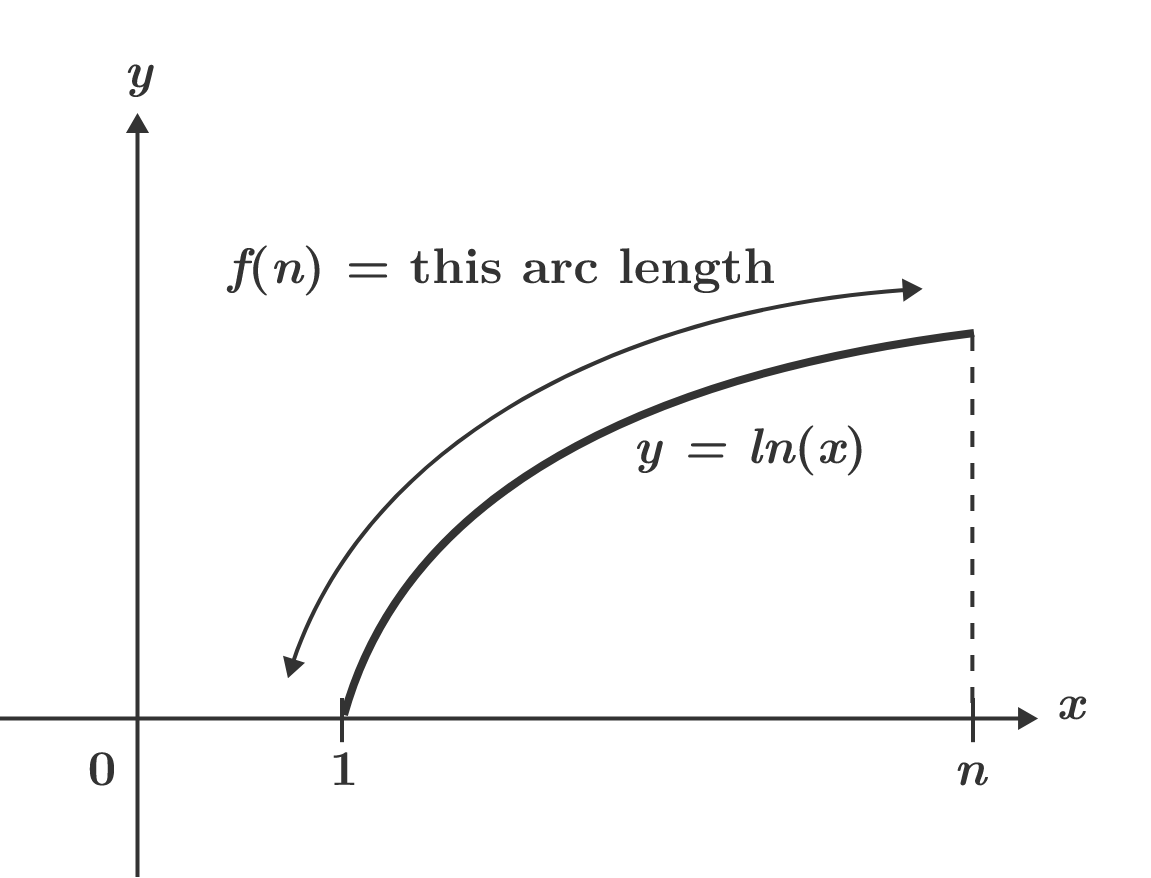# Arc Length of $\ln\left(x\right)$

Let $f(n)$ denote the arc length of the curve $y=\ln\left(x\right)$ in the interval $x\in\left [1,n\right ]$. Find $\lim_{n\to\infty}\big(n-f(n)\big).$ If your answer is of the form $\ln\left(\sqrt{a}-b\right)+\sqrt{c}-d$, where $a$, $b$, $c$, and $d$ are non-negative integers and $a$ and $c$ are not perfect squares, find $a+b+c+d$.×